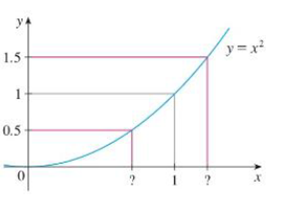Chapter 2.4, Problem 4E

Chapter
Section
Textbook Problem

Use the given graph of f(x) =x2 to find a number δ such thatif |x –1|< δ then |x2– 1 | < 1 2To determine

To find: The number δ by using the graph.

Explanation

Definition used:

“Let f be a function defined on some open interval that contains the number a, except possibly at a itself. Then, the limit of f(x) as x approaches a is L, limxaf(x)=L if for every number ε>0 there is a number δ>0 such that if 0<|xa|<δ then |f(x)L|<ε”.

Given:

The graph of the function is f(x)=x2.

There exists a number δ such that |x21|<12 whenever |x1|<δ.

From the graph, it is observed that the values of a=1, L=1, y=x2.

Calculation:

Obtain the value of δ by using the graph of f(x)=x2.

By the definition of ε and δ, it is enough to find a number δ such that |x21|<12 whenever |x1|<δ.

The leftmost question mark is denoted as 1δ1 and the rightmost question mark is denoted as 1+δ2.

From the graph, at x=1δ1 is the solution of y=0.5. That is,

x2=0.5x=0.5x0.7071

Thus, the value of the leftmost question mark or the distance from x=1 to the left endpoint is 1δ1=0

Still sussing out bartleby?

Check out a sample textbook solution.

See a sample solution

The Solution to Your Study Problems

Bartleby provides explanations to thousands of textbook problems written by our experts, many with advanced degrees!

Get Started